Next: Adaptive filtering from the Up: Noise reduction from the Previous: The Wiener-like filtering in

Hierarchical Wiener filtering

In the above process, we do not use the information between the wavelet coefficients at different scales. We modify the previous algorithm by introducing a prediction wh of the wavelet coefficient from the upper scale. This prediction could be determined from the regression  between the two scales but better results are obtained when we only set wh to Wi+1. Between the expectation coefficient Wi and the prediction, a dispersion exists where we assume that it is a Gaussian distribution:(14.84)

The relation which gives the coefficient Wi knowing wi and wh is: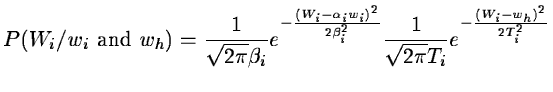(14.85)

with: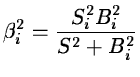(14.86)

and: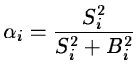(14.87)

This follows a Gaussian distribution with a mathematical expectation: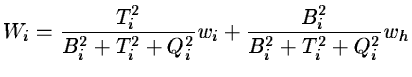(14.88)

with: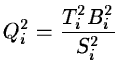(14.89)

Wi is the barycentre of the three values wi, wh, 0 with the weights Ti2, Bi2, Qi2. The particular cases are:
• If the noise is large (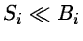) and even if the correlation between the two scales is good (Ti is low), we get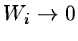.
• if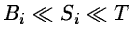then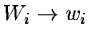.
• if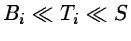then.
• if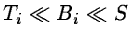then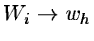.

At each scale, by changing all the wavelet coefficients wi of the plane by the estimate value Wi, we get a Hierarchical Wiener Filter. The algorithm is:

1.
Compute the wavelet transform of the data. We get wi.
2.
Estimate the standard deviation of the noise B0 of the first plane from the histogram of w0.
3.
Set i to the index associated with the last plane: i = n
4.
Estimate the standard deviation of the noise Bi from B0.
5.
Si2 = si2 - Bi2 where si2 is the variance of wi
6.
Set wh to Wi+1 and compute the standard deviation Tiof wi - wh.
7.8.
i = i - 1. If i > 0 go to 4
9.
Reconstruct the pictureNext: Adaptive filtering from the Up: Noise reduction from the Previous: The Wiener-like filtering in
Petra Nass
1999-06-15